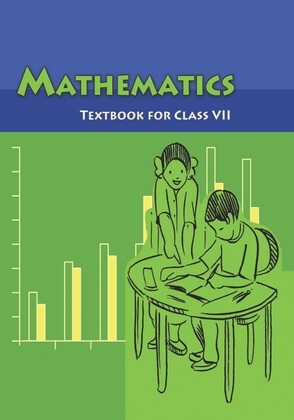•COMPARING QUANTITIES Amount of change = decrease in the number of illiterate persons = 150 – 100 = 50 lakhs Therefore, the percentage of decrease amount of change 50 1 original amount 150 3 8.5 PRICES RELATED TO AN ITEM OR BUYING AND SELLING I bought it for ` 600and will sell it for ` 610 The buying price of any item is known as its cost price. It is written in short as CP. The price at which you sell is known as the selling price or in short SP. What would you say is better, to you sell the item at a lower price, same price or higher price than your buying price? You can decide whether the sale was profitable or not depending on the CP and SP. If CP < SP then you made a profit = SP – CP. If CP = SP then you are in a no profit no loss situation. If CP > SP then you have a loss = CP – SP. Let us try to interpret the statements related to prices of items. • A toy bought for ` 72 is sold at ` 80. • AT-shirt bought for ` 120 is sold at ` 100. • A cycle bought for ` 800 is sold for ` 940. Let us consider the first statement. The buying price (or CP) is ` 72 and the selling price (or SP) is ` 80. This means SP is more than CP. Hence profit made = SP – CP = ` 80 – ` 72 = ` 8 Now try interpreting the remaining statements in a similar way. 8.5.1 Profit or Loss as a Percentage The profit or loss can be converted to a percentage. It is always calculated on the CP. For the above examples, we can find the profit % or loss %. Let us consider the example related to the toy. We have CP = ` 72, SP = ` 80, Profit = ` 8. To find the percentage of profit, Neha and Shekhar have used the following methods. COMPARING QUANTITIES EXAMPLE 19 Selling price of a toy car is ` 540. If the profit made by shopkeeper is 20%, what is the cost price of this toy? SOLUTION We are given that SP= `540 and the Profit = 20%.We need to find the CP. 8.6 CHARGE GIVEN ON BORROWED MONEY OR SIMPLE INTEREST Sohini said that they were going to buy a new scooter. Mohan asked her whether they had the money to buy it. Sohini said her father was going to take a loan from a bank. The money you borrow is known as sum borrowed or principal. This money would be used by the borrower for some time before it is returned. For keeping this money for some time the borrower has to pay some extra money to the bank. This is known as Interest. You can find the amount you have to pay at the end of the year by adding the sum borrowed and the interest. That is, Amount = Principal + Interest. Interest is generally given in per cent for a period of one year. It is written as say 10% per year or per annum or in short as 10% p.a. (per annum). 10% p.a. means on every ` 100 borrowed, ` 10 is the interest you have to pay for one year. Let us take an example and see how this works. EXAMPLE 20 Anita takes a loan of ` 5,000 at 15% per year as rate of interest. Find the interest she has to pay at the end of one year.